{{ message }}
/ imgaug Public

Image augmentation for machine learning experiments.

# aleju/imgaug

Switch branches/tags
Could not load branches
Nothing to show

## Latest commit

```This patch fixes a deprecation warning in
`Affine` that would be caused when providing
boolean images and `order != 0`.```
`0101108`

## Files

Failed to load latest commit information.
Type
Name
Commit time

# imgaug

This python library helps you with augmenting images for your machine learning projects. It converts a set of input images into a new, much larger set of slightly altered images.

More (strong) example augmentations of one input image: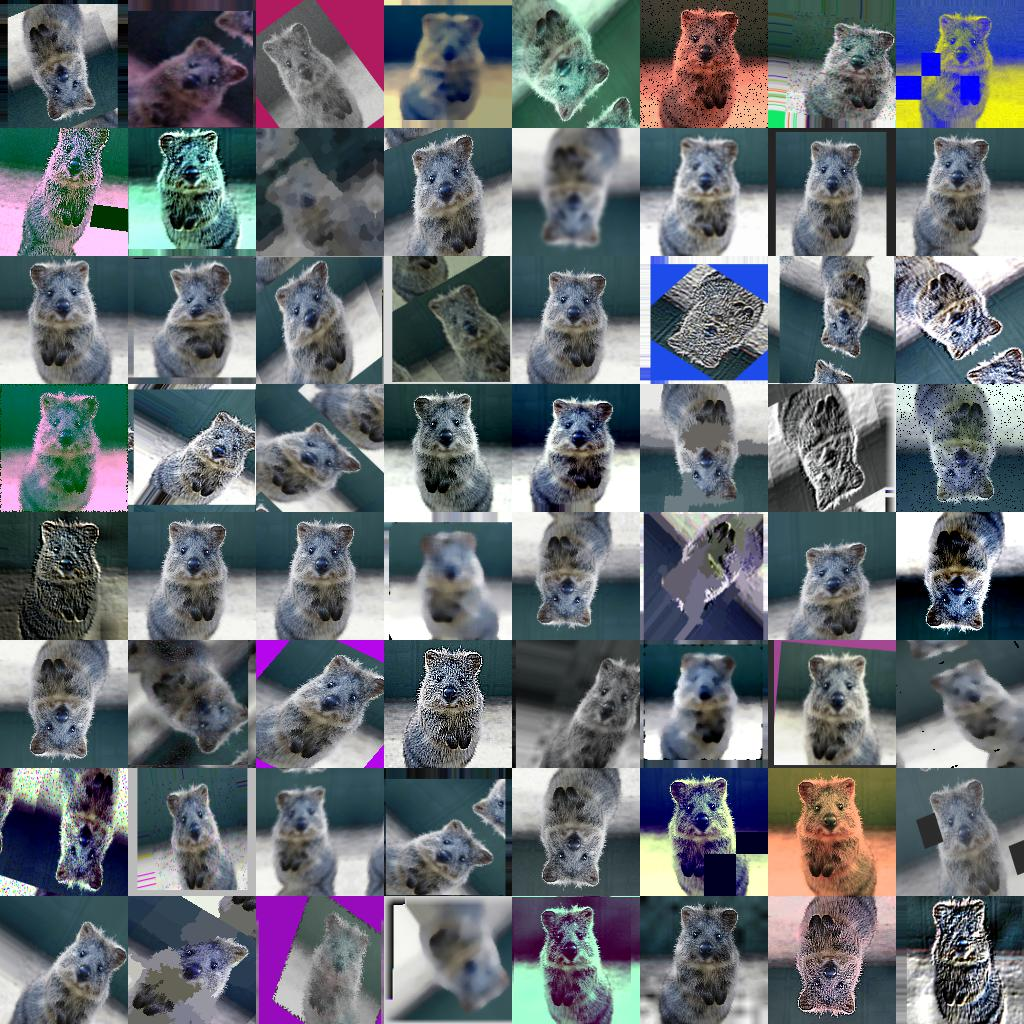## Installation

The library supports python 2.7 and 3.4+.

### Installation: Anaconda

To install the library in anaconda, perform the following commands:

```conda config --add channels conda-forge
conda install imgaug```

You can deinstall the library again via `conda remove imgaug`.

### Installation: pip

Then install imgaug either via pypi (can lag behind the github version):

`pip install imgaug`

or install the latest version directly from github:

`pip install git+https://github.com/aleju/imgaug.git`

To deinstall the library, just execute `pip uninstall imgaug`.

## Documentation

Example jupyter notebooks:

More notebooks: imgaug-doc/notebooks.

More RTD documentation: imgaug.readthedocs.io.

All documentation related files of this project are hosted in the repository imgaug-doc.

## Recent Changes

• 0.4.0: Added new augmenters, changed backend to batchwise augmentation, support for numpy 1.18 and python 3.8.
• 0.3.0: Reworked segmentation map augmentation, adapted to numpy 1.17+ random number sampling API, several new augmenters.
• 0.2.9: Added polygon augmentation, added line string augmentation, simplified augmentation interface.
• 0.2.8: Improved performance, dtype support and multicore augmentation.

See changelogs/ for more details.

## Example Images

The images below show examples for most augmentation techniques.

Values written in the form `(a, b)` denote a uniform distribution, i.e. the value is randomly picked from the interval `[a, b]`. Line strings are supported by (almost) all augmenters, but are not explicitly visualized here.

 meta Identity ChannelShuffle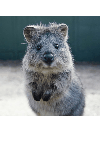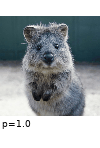See also: Sequential, SomeOf, OneOf, Sometimes, WithChannels, Lambda, AssertLambda, AssertShape, RemoveCBAsByOutOfImageFraction, ClipCBAsToImagePlanes arithmetic Add Add(per_channel=True) AdditiveGaussianNoise AdditiveGaussianNoise(per_channel=True) Multiply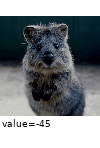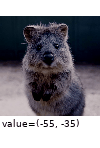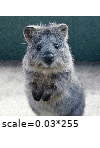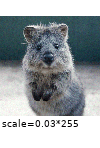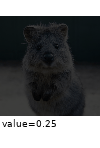Cutout Dropout CoarseDropout(p=0.2) CoarseDropout(p=0.2, per_channel=True) Dropout2d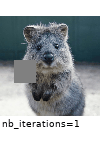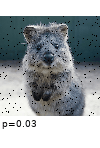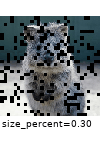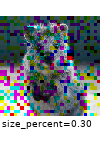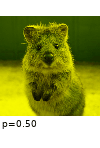SaltAndPepper CoarseSaltAndPepper(p=0.2) Invert Solarize JpegCompression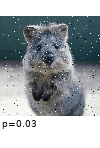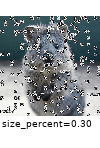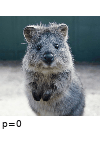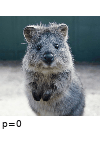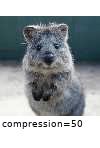See also: AddElementwise, AdditiveLaplaceNoise, AdditivePoissonNoise, MultiplyElementwise, TotalDropout, ReplaceElementwise, ImpulseNoise, Salt, Pepper, CoarseSalt, CoarsePepper, Solarize artistic Cartoon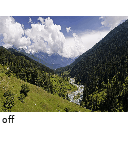blend BlendAlphawith EdgeDetect(1.0) BlendAlphaSimplexNoisewith EdgeDetect(1.0) BlendAlphaFrequencyNoisewith EdgeDetect(1.0) BlendAlphaSomeColorswith RemoveSaturation(1.0) BlendAlphaRegularGridwith Multiply((0.0, 0.5))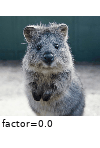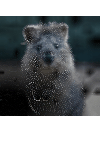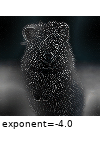See also: BlendAlphaMask, BlendAlphaElementwise, BlendAlphaVerticalLinearGradient, BlendAlphaHorizontalLinearGradient, BlendAlphaSegMapClassIds, BlendAlphaBoundingBoxes, BlendAlphaCheckerboard, SomeColorsMaskGen, HorizontalLinearGradientMaskGen, VerticalLinearGradientMaskGen, RegularGridMaskGen, CheckerboardMaskGen, SegMapClassIdsMaskGen, BoundingBoxesMaskGen, InvertMaskGen blur GaussianBlur AverageBlur MedianBlur BilateralBlur(sigma_color=250,sigma_space=250) MotionBlur(angle=0)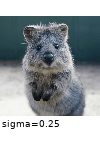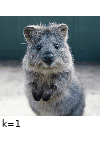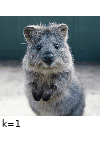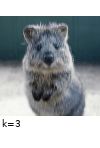MotionBlur(k=5) MeanShiftBlur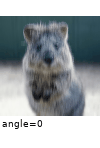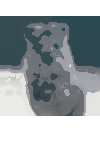collections RandAugment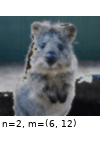color MultiplyAndAddToBrightness MultiplyHueAndSaturation MultiplyHue MultiplySaturation AddToHueAndSaturation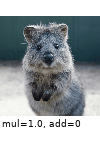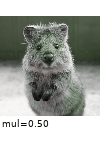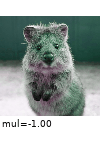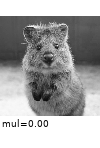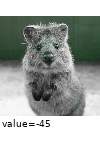Grayscale RemoveSaturation ChangeColorTemperature KMeansColorQuantization(to_colorspace=RGB) UniformColorQuantization(to_colorspace=RGB)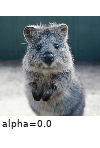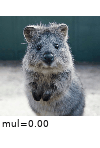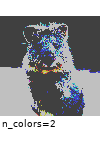See also: WithColorspace, WithBrightnessChannels, MultiplyBrightness, AddToBrightness, WithHueAndSaturation, AddToHue, AddToSaturation, ChangeColorspace, Posterize contrast GammaContrast GammaContrast(per_channel=True) SigmoidContrast(cutoff=0.5) SigmoidContrast(gain=10) LogContrast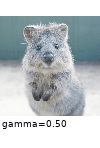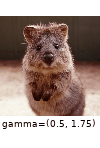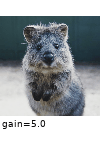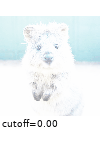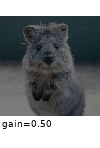LinearContrast AllChannels-HistogramEqualization HistogramEqualization AllChannelsCLAHE CLAHE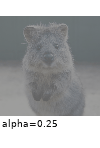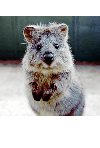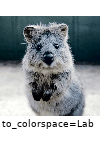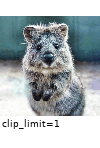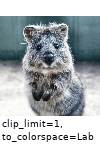See also: Equalize convolutional Sharpen(alpha=1) Emboss(alpha=1) EdgeDetect DirectedEdgeDetect(alpha=1)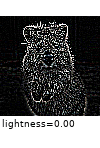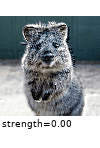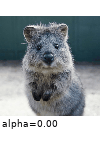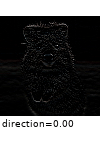See also: Convolve debug See also: SaveDebugImageEveryNBatches edges Canny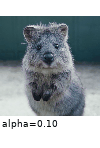flip Fliplr Flipud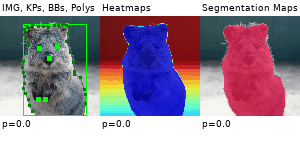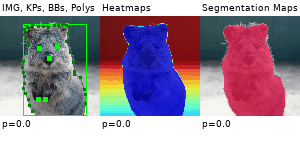See also: HorizontalFlip, VerticalFlip geometric Affine Affine: Modes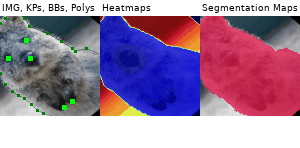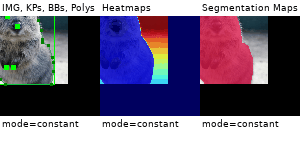Affine: cval PiecewiseAffine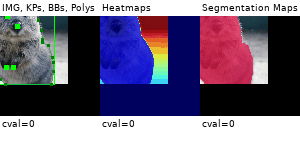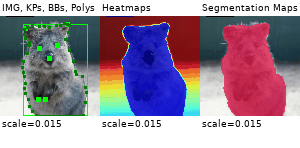PerspectiveTransform ElasticTransformation(sigma=1.0)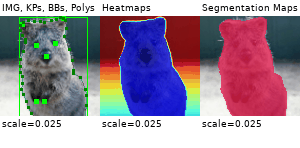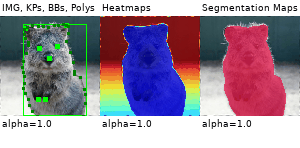ElasticTransformation(sigma=4.0) Rot90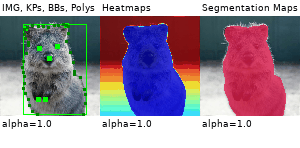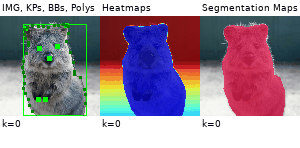WithPolarWarping+Affine Jigsaw(5x5 grid)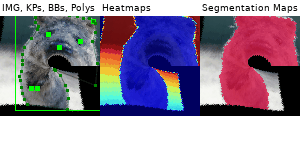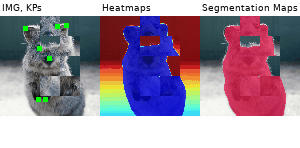See also: ScaleX, ScaleY, TranslateX, TranslateY, Rotate imgcorruptlike GlassBlur DefocusBlur ZoomBlur Snow Spatter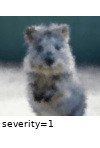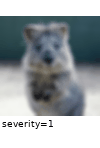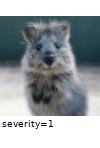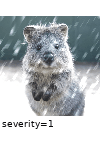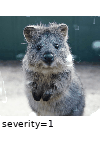See also: GaussianNoise, ShotNoise, ImpulseNoise, SpeckleNoise, GaussianBlur, MotionBlur, Fog, Frost, Contrast, Brightness, Saturate, JpegCompression, Pixelate, ElasticTransform pillike Autocontrast EnhanceColor EnhanceSharpness FilterEdgeEnhanceMore FilterContour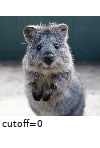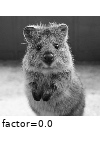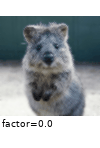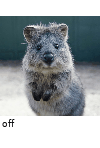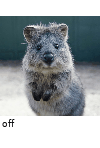See also: Solarize, Posterize, Equalize, EnhanceContrast, EnhanceBrightness, FilterBlur, FilterSmooth, FilterSmoothMore, FilterEdgeEnhance, FilterFindEdges, FilterEmboss, FilterSharpen, FilterDetail, Affine pooling AveragePooling MaxPooling MinPooling MedianPooling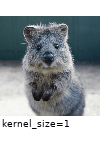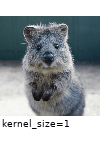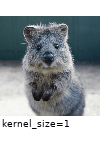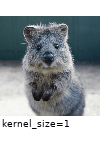segmentation Superpixels(p_replace=1) Superpixels(n_segments=100) UniformVoronoi RegularGridVoronoi: rows/cols(p_drop_points=0) RegularGridVoronoi: p_drop_points(n_rows=n_cols=30)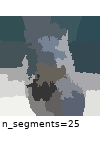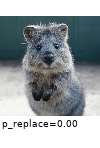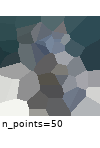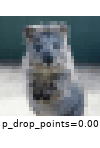RegularGridVoronoi: p_replace(n_rows=n_cols=16)See also: Voronoi, RelativeRegularGridVoronoi, RegularGridPointsSampler, RelativeRegularGridPointsSampler, DropoutPointsSampler, UniformPointsSampler, SubsamplingPointsSampler size CropAndPad Crop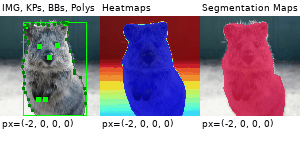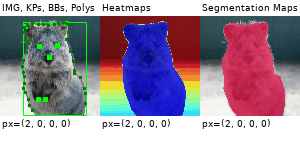Pad PadToFixedSize(height'=height+32,width'=width+32)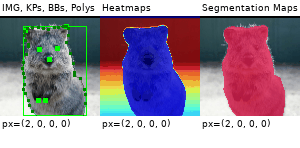CropToFixedSize(height'=height-32,width'=width-32)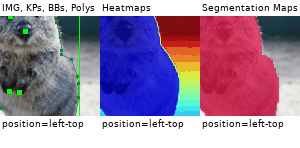See also: Resize, CropToMultiplesOf, PadToMultiplesOf, CropToPowersOf, PadToPowersOf, CropToAspectRatio, PadToAspectRatio, CropToSquare, PadToSquare, CenterCropToFixedSize, CenterPadToFixedSize, CenterCropToMultiplesOf, CenterPadToMultiplesOf, CenterCropToPowersOf, CenterPadToPowersOf, CenterCropToAspectRatio, CenterPadToAspectRatio, CenterCropToSquare, CenterPadToSquare, KeepSizeByResize weather FastSnowyLandscape(lightness_multiplier=2.0) Clouds Fog Snowflakes Rain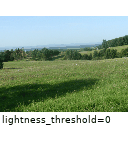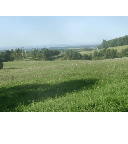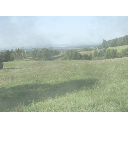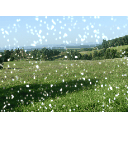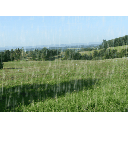See also: CloudLayer, SnowflakesLayer, RainLayer

## Code Examples

### Example: Simple Training Setting

A standard machine learning situation. Train on batches of images and augment each batch via crop, horizontal flip ("Fliplr") and gaussian blur:

```import numpy as np
import imgaug.augmenters as iaa

# dummy function, implement this
# Return a numpy array of shape (N, height, width, #channels)
# or a list of (height, width, #channels) arrays (may have different image
# sizes).
# Images should be in RGB for colorspace augmentations.
# (cv2.imread() returns BGR!)
# Images should usually be in uint8 with values from 0-255.
return np.zeros((128, 32, 32, 3), dtype=np.uint8) + (batch_idx % 255)

def train_on_images(images):
# dummy function, implement this
pass

# Pipeline:
# (1) Crop images from each side by 1-16px, do not resize the results
#     images back to the input size. Keep them at the cropped size.
# (2) Horizontally flip 50% of the images.
# (3) Blur images using a gaussian kernel with sigma between 0.0 and 3.0.
seq = iaa.Sequential([
iaa.Crop(px=(1, 16), keep_size=False),
iaa.Fliplr(0.5),
iaa.GaussianBlur(sigma=(0, 3.0))
])

for batch_idx in range(100):
images_aug = seq(images=images)  # done by the library
train_on_images(images_aug)```

### Example: Very Complex Augmentation Pipeline

Apply a very heavy augmentation pipeline to images (used to create the image at the very top of this readme):

```import numpy as np
import imgaug as ia
import imgaug.augmenters as iaa

# random example images
images = np.random.randint(0, 255, (16, 128, 128, 3), dtype=np.uint8)

# Sometimes(0.5, ...) applies the given augmenter in 50% of all cases,
# e.g. Sometimes(0.5, GaussianBlur(0.3)) would blur roughly every second image.
sometimes = lambda aug: iaa.Sometimes(0.5, aug)

# Define our sequence of augmentation steps that will be applied to every image
# All augmenters with per_channel=0.5 will sample one value _per image_
# in 50% of all cases. In all other cases they will sample new values
# _per channel_.

seq = iaa.Sequential(
[
# apply the following augmenters to most images
iaa.Fliplr(0.5), # horizontally flip 50% of all images
iaa.Flipud(0.2), # vertically flip 20% of all images
# crop images by -5% to 10% of their height/width
percent=(-0.05, 0.1),
)),
sometimes(iaa.Affine(
scale={"x": (0.8, 1.2), "y": (0.8, 1.2)}, # scale images to 80-120% of their size, individually per axis
translate_percent={"x": (-0.2, 0.2), "y": (-0.2, 0.2)}, # translate by -20 to +20 percent (per axis)
rotate=(-45, 45), # rotate by -45 to +45 degrees
shear=(-16, 16), # shear by -16 to +16 degrees
order=[0, 1], # use nearest neighbour or bilinear interpolation (fast)
cval=(0, 255), # if mode is constant, use a cval between 0 and 255
mode=ia.ALL # use any of scikit-image's warping modes (see 2nd image from the top for examples)
)),
# execute 0 to 5 of the following (less important) augmenters per image
# don't execute all of them, as that would often be way too strong
iaa.SomeOf((0, 5),
[
sometimes(iaa.Superpixels(p_replace=(0, 1.0), n_segments=(20, 200))), # convert images into their superpixel representation
iaa.OneOf([
iaa.GaussianBlur((0, 3.0)), # blur images with a sigma between 0 and 3.0
iaa.AverageBlur(k=(2, 7)), # blur image using local means with kernel sizes between 2 and 7
iaa.MedianBlur(k=(3, 11)), # blur image using local medians with kernel sizes between 2 and 7
]),
iaa.Sharpen(alpha=(0, 1.0), lightness=(0.75, 1.5)), # sharpen images
iaa.Emboss(alpha=(0, 1.0), strength=(0, 2.0)), # emboss images
# search either for all edges or for directed edges,
# blend the result with the original image using a blobby mask
iaa.SimplexNoiseAlpha(iaa.OneOf([
iaa.EdgeDetect(alpha=(0.5, 1.0)),
iaa.DirectedEdgeDetect(alpha=(0.5, 1.0), direction=(0.0, 1.0)),
])),
iaa.AdditiveGaussianNoise(loc=0, scale=(0.0, 0.05*255), per_channel=0.5), # add gaussian noise to images
iaa.OneOf([
iaa.Dropout((0.01, 0.1), per_channel=0.5), # randomly remove up to 10% of the pixels
iaa.CoarseDropout((0.03, 0.15), size_percent=(0.02, 0.05), per_channel=0.2),
]),
iaa.Invert(0.05, per_channel=True), # invert color channels
iaa.Add((-10, 10), per_channel=0.5), # change brightness of images (by -10 to 10 of original value)
iaa.AddToHueAndSaturation((-20, 20)), # change hue and saturation
# either change the brightness of the whole image (sometimes
# per channel) or change the brightness of subareas
iaa.OneOf([
iaa.Multiply((0.5, 1.5), per_channel=0.5),
iaa.FrequencyNoiseAlpha(
exponent=(-4, 0),
first=iaa.Multiply((0.5, 1.5), per_channel=True),
second=iaa.LinearContrast((0.5, 2.0))
)
]),
iaa.LinearContrast((0.5, 2.0), per_channel=0.5), # improve or worsen the contrast
iaa.Grayscale(alpha=(0.0, 1.0)),
sometimes(iaa.ElasticTransformation(alpha=(0.5, 3.5), sigma=0.25)), # move pixels locally around (with random strengths)
sometimes(iaa.PiecewiseAffine(scale=(0.01, 0.05))), # sometimes move parts of the image around
sometimes(iaa.PerspectiveTransform(scale=(0.01, 0.1)))
],
random_order=True
)
],
random_order=True
)
images_aug = seq(images=images)```

### Example: Augment Images and Keypoints

Augment images and keypoints/landmarks on the same images:

```import numpy as np
import imgaug.augmenters as iaa

images = np.zeros((2, 128, 128, 3), dtype=np.uint8)  # two example images
images[:, 64, 64, :] = 255
points = [
[(10.5, 20.5)],  # points on first image
[(50.5, 50.5), (60.5, 60.5), (70.5, 70.5)]  # points on second image
]

seq = iaa.Sequential([
iaa.Affine(translate_px={"x": (1, 5)})
])

# augment keypoints and images
images_aug, points_aug = seq(images=images, keypoints=points)

print("Image 1 center", np.argmax(images_aug[0, 64, 64:64+6, 0]))
print("Image 2 center", np.argmax(images_aug[1, 64, 64:64+6, 0]))
print("Points 1", points_aug)
print("Points 2", points_aug)```

Note that all coordinates in `imgaug` are subpixel-accurate, which is why `x=0.5, y=0.5` denotes the center of the top left pixel.

### Example: Augment Images and Bounding Boxes

```import numpy as np
import imgaug as ia
import imgaug.augmenters as iaa

images = np.zeros((2, 128, 128, 3), dtype=np.uint8)  # two example images
images[:, 64, 64, :] = 255
bbs = [
[ia.BoundingBox(x1=10.5, y1=15.5, x2=30.5, y2=50.5)],
[ia.BoundingBox(x1=10.5, y1=20.5, x2=50.5, y2=50.5),
ia.BoundingBox(x1=40.5, y1=75.5, x2=70.5, y2=100.5)]
]

seq = iaa.Sequential([
iaa.Affine(translate_px={"x": (1, 5)})
])

images_aug, bbs_aug = seq(images=images, bounding_boxes=bbs)```

### Example: Augment Images and Polygons

```import numpy as np
import imgaug as ia
import imgaug.augmenters as iaa

images = np.zeros((2, 128, 128, 3), dtype=np.uint8)  # two example images
images[:, 64, 64, :] = 255
polygons = [
[ia.Polygon([(10.5, 10.5), (50.5, 10.5), (50.5, 50.5)])],
[ia.Polygon([(0.0, 64.5), (64.5, 0.0), (128.0, 128.0), (64.5, 128.0)])]
]

seq = iaa.Sequential([
iaa.Affine(translate_px={"x": (1, 5)})
])

images_aug, polygons_aug = seq(images=images, polygons=polygons)```

### Example: Augment Images and LineStrings

LineStrings are similar to polygons, but are not closed, may intersect with themselves and don't have an inner area.

```import numpy as np
import imgaug as ia
import imgaug.augmenters as iaa

images = np.zeros((2, 128, 128, 3), dtype=np.uint8)  # two example images
images[:, 64, 64, :] = 255
ls = [
[ia.LineString([(10.5, 10.5), (50.5, 10.5), (50.5, 50.5)])],
[ia.LineString([(0.0, 64.5), (64.5, 0.0), (128.0, 128.0), (64.5, 128.0),
(128.0, 0.0)])]
]

seq = iaa.Sequential([
iaa.Affine(translate_px={"x": (1, 5)})
])

images_aug, ls_aug = seq(images=images, line_strings=ls)```

### Example: Augment Images and Heatmaps

Heatmaps are dense float arrays with values between `0.0` and `1.0`. They can be used e.g. when training models to predict facial landmark locations. Note that the heatmaps here have lower height and width than the images. `imgaug` handles that case automatically. The crop pixel amounts will be halved for the heatmaps.

```import numpy as np
import imgaug.augmenters as iaa

# Standard scenario: You have N RGB-images and additionally 21 heatmaps per
# image. You want to augment each image and its heatmaps identically.
images = np.random.randint(0, 255, (16, 128, 128, 3), dtype=np.uint8)
heatmaps = np.random.random(size=(16, 64, 64, 1)).astype(np.float32)

seq = iaa.Sequential([
iaa.GaussianBlur((0, 3.0)),
iaa.Affine(translate_px={"x": (-40, 40)}),
iaa.Crop(px=(0, 10))
])

images_aug, heatmaps_aug = seq(images=images, heatmaps=heatmaps)```

### Example: Augment Images and Segmentation Maps

This is similar to heatmaps, but the dense arrays have dtype `int32`. Operations such as resizing will automatically use nearest neighbour interpolation.

```import numpy as np
import imgaug.augmenters as iaa

# Standard scenario: You have N=16 RGB-images and additionally one segmentation
# map per image. You want to augment each image and its heatmaps identically.
images = np.random.randint(0, 255, (16, 128, 128, 3), dtype=np.uint8)
segmaps = np.random.randint(0, 10, size=(16, 64, 64, 1), dtype=np.int32)

seq = iaa.Sequential([
iaa.GaussianBlur((0, 3.0)),
iaa.Affine(translate_px={"x": (-40, 40)}),
iaa.Crop(px=(0, 10))
])

images_aug, segmaps_aug = seq(images=images, segmentation_maps=segmaps)```

### Example: Visualize Augmented Images

Quickly show example results of your augmentation sequence:

```import numpy as np
import imgaug.augmenters as iaa

images = np.random.randint(0, 255, (16, 128, 128, 3), dtype=np.uint8)
seq = iaa.Sequential([iaa.Fliplr(0.5), iaa.GaussianBlur((0, 3.0))])

# Show an image with 8*8 augmented versions of image 0 and 8*8 augmented
# versions of image 1. Identical augmentations will be applied to
# image 0 and 1.
seq.show_grid([images, images], cols=8, rows=8)```

### Example: Visualize Augmented Non-Image Data

`imgaug` contains many helper function, among these functions to quickly visualize augmented non-image results, such as bounding boxes or heatmaps.

```import numpy as np
import imgaug as ia

image = np.zeros((64, 64, 3), dtype=np.uint8)

# points
kps = [ia.Keypoint(x=10.5, y=20.5), ia.Keypoint(x=60.5, y=60.5)]
kpsoi = ia.KeypointsOnImage(kps, shape=image.shape)
image_with_kps = kpsoi.draw_on_image(image, size=7, color=(0, 0, 255))
ia.imshow(image_with_kps)

# bbs
bbsoi = ia.BoundingBoxesOnImage([
ia.BoundingBox(x1=10.5, y1=20.5, x2=50.5, y2=30.5)
], shape=image.shape)
image_with_bbs = bbsoi.draw_on_image(image)
image_with_bbs = ia.BoundingBox(
x1=50.5, y1=10.5, x2=100.5, y2=16.5
).draw_on_image(image_with_bbs, color=(255, 0, 0), size=3)
ia.imshow(image_with_bbs)

# polygons
psoi = ia.PolygonsOnImage([
ia.Polygon([(10.5, 20.5), (50.5, 30.5), (10.5, 50.5)])
], shape=image.shape)
image_with_polys = psoi.draw_on_image(
image, alpha_points=0, alpha_face=0.5, color_lines=(255, 0, 0))
ia.imshow(image_with_polys)

# heatmaps
hms = ia.HeatmapsOnImage(np.random.random(size=(32, 32, 1)).astype(np.float32),
shape=image.shape)
image_with_hms = hms.draw_on_image(image)
ia.imshow(image_with_hms)```

LineStrings and segmentation maps support similar methods as shown above.

### Example: Using Augmenters Only Once

While the interface is adapted towards re-using instances of augmenters many times, you are also free to use them only once. The overhead to instantiate the augmenters each time is usually negligible.

```from imgaug import augmenters as iaa
import numpy as np

images = np.random.randint(0, 255, (16, 128, 128, 3), dtype=np.uint8)

# always horizontally flip each input image
images_aug = iaa.Fliplr(1.0)(images=images)

# vertically flip each input image with 90% probability
images_aug = iaa.Flipud(0.9)(images=images)

# blur 50% of all images using a gaussian kernel with a sigma of 3.0
images_aug = iaa.Sometimes(0.5, iaa.GaussianBlur(3.0))(images=images)```

### Example: Multicore Augmentation

Images can be augmented in background processes using the method `augment_batches(batches, background=True)`, where `batches` is a list/generator of imgaug.augmentables.batches.UnnormalizedBatch or imgaug.augmentables.batches.Batch. The following example augments a list of image batches in the background:

```import skimage.data
import imgaug as ia
import imgaug.augmenters as iaa
from imgaug.augmentables.batches import UnnormalizedBatch

# Number of batches and batch size for this example
nb_batches = 10
batch_size = 32

# Example augmentation sequence to run in the background
augseq = iaa.Sequential([
iaa.Fliplr(0.5),
iaa.CoarseDropout(p=0.1, size_percent=0.1)
])

# For simplicity, we use the same image here many times
astronaut = skimage.data.astronaut()
astronaut = ia.imresize_single_image(astronaut, (64, 64))

# Make batches out of the example image (here: 10 batches, each 32 times
# the example image)
batches = []
for _ in range(nb_batches):
batches.append(UnnormalizedBatch(images=[astronaut] * batch_size))

# Show the augmented images.
# Note that augment_batches() returns a generator.
for images_aug in augseq.augment_batches(batches, background=True):
ia.imshow(ia.draw_grid(images_aug.images_aug, cols=8))```

If you need more control over the background augmentation, e.g. to set seeds, control the number of used CPU cores or constraint the memory usage, see the corresponding multicore augmentation notebook or the API about Augmenter.pool() and imgaug.multicore.Pool.

### Example: Probability Distributions as Parameters

Most augmenters support using tuples `(a, b)` as a shortcut to denote `uniform(a, b)` or lists `[a, b, c]` to denote a set of allowed values from which one will be picked randomly. If you require more complex probability distributions (e.g. gaussians, truncated gaussians or poisson distributions) you can use stochastic parameters from `imgaug.parameters`:

```import numpy as np
from imgaug import augmenters as iaa
from imgaug import parameters as iap

images = np.random.randint(0, 255, (16, 128, 128, 3), dtype=np.uint8)

# Blur by a value sigma which is sampled from a uniform distribution
# of range 10.1 <= x < 13.0.
# The convenience shortcut for this is: GaussianBlur((10.1, 13.0))
blurer = iaa.GaussianBlur(10 + iap.Uniform(0.1, 3.0))
images_aug = blurer(images=images)

# Blur by a value sigma which is sampled from a gaussian distribution
# N(1.0, 0.1), i.e. sample a value that is usually around 1.0.
# Clip the resulting value so that it never gets below 0.1 or above 3.0.
blurer = iaa.GaussianBlur(iap.Clip(iap.Normal(1.0, 0.1), 0.1, 3.0))
images_aug = blurer(images=images)```

There are many more probability distributions in the library, e.g. truncated gaussian distribution, poisson distribution or beta distribution.

### Example: WithChannels

Apply an augmenter only to specific image channels:

```import numpy as np
import imgaug.augmenters as iaa

# fake RGB images
images = np.random.randint(0, 255, (16, 128, 128, 3), dtype=np.uint8)

# add a random value from the range (-30, 30) to the first two channels of
# input images (e.g. to the R and G channels)
aug = iaa.WithChannels(
channels=[0, 1],
)

images_aug = aug(images=images)```

## Citation

If this library has helped you during your research, feel free to cite it:

```@misc{imgaug,
author = {Jung, Alexander B.
and Crall, Jon
and Tanaka, Satoshi
and Graving, Jake
and Reinders, Christoph
and Banerjee, Joy
and Vecsei, Gábor
and Rui, Zheng
and Borovec, Jirka
and Vallentin, Christian
and Zhydenko, Semen
and Pfeiffer, Kilian
and Cook, Ben
and Fernández, Ismael
and De Rainville, François-Michel
and Weng, Chi-Hung
and Ayala-Acevedo, Abner
and Meudec, Raphael
and Laporte, Matias
and others},
title = {{imgaug}},
howpublished = {\url{https://github.com/aleju/imgaug}},
year = {2020},
note = {Online; accessed 01-Feb-2020}
}```

Image augmentation for machine learning experiments.

0.4.0 Latest
Feb 6, 2020

## Packages 0

No packages published